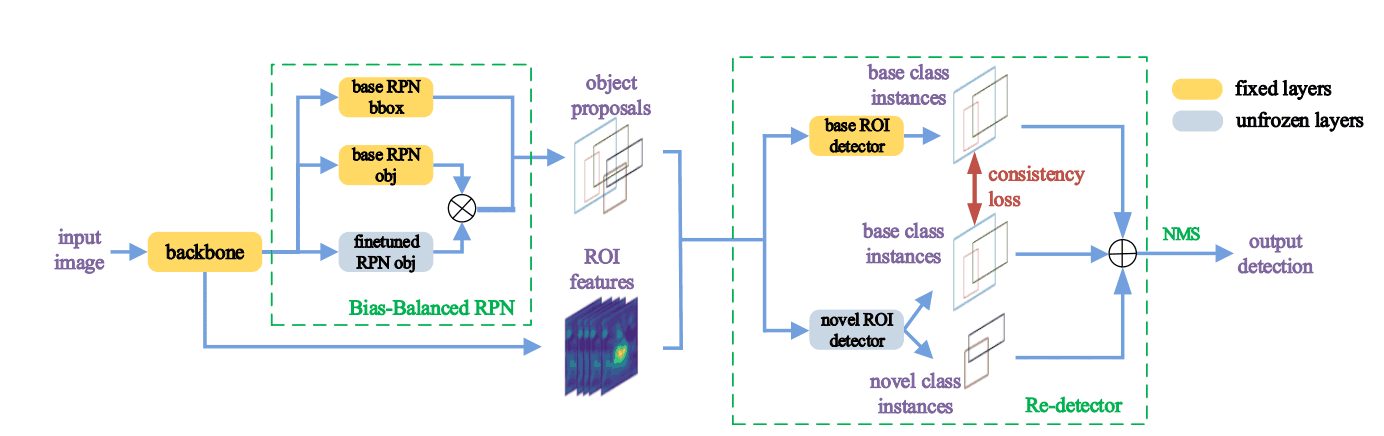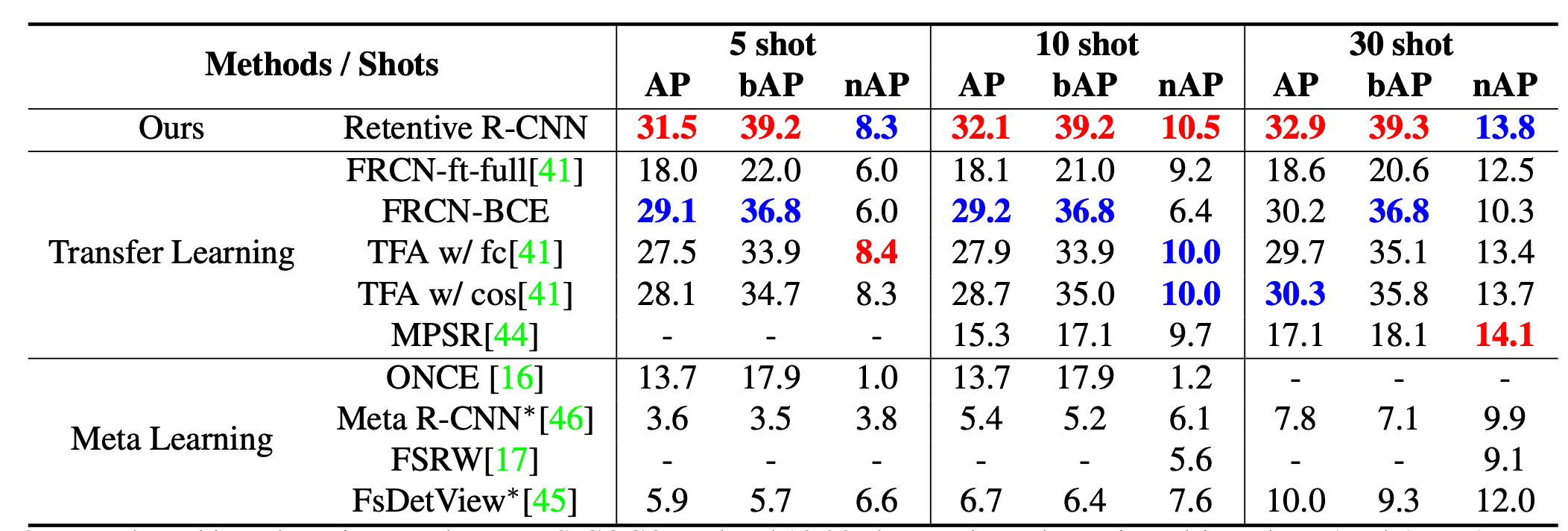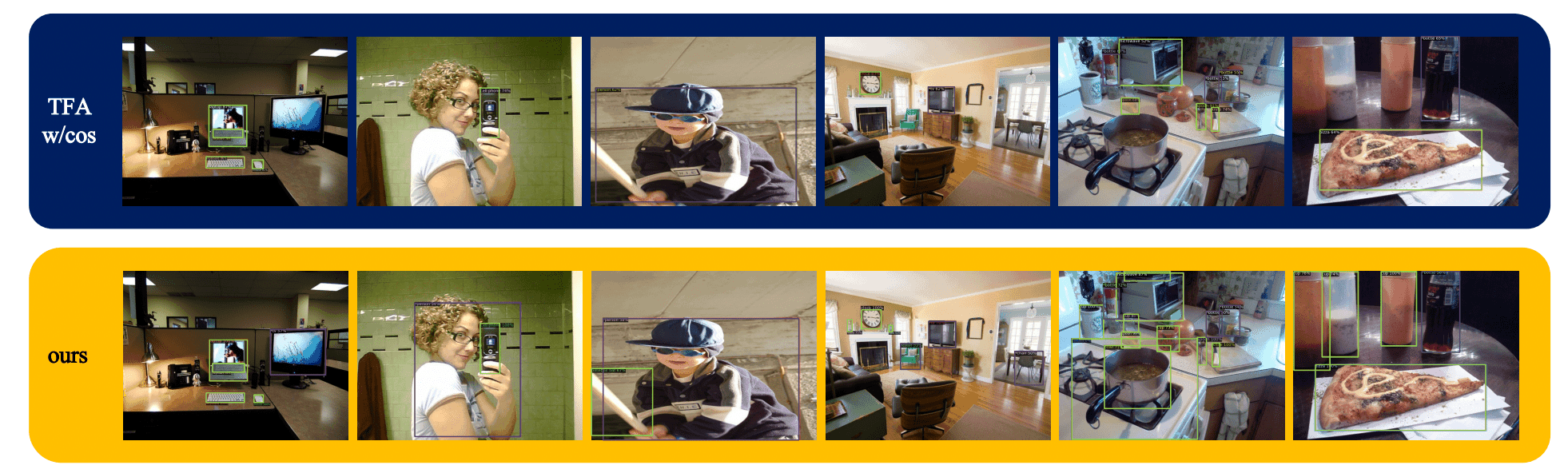# Catch up on the latest AI articles# Few-shot Object Detection That Does Not Forget The Base Class Either

3 main points
✔️ We developed an object detection model, Retentive R-CNN, which can detect the few-shot class without forgetting the learned base class information.
✔️ Retentive R-CNN recorded SOTA on the benchmark of few-shot detection.
✔️ The performance did not drop at all in base class detection.

written by Zhibo FanYuchen MaZeming LiJian Sun
(Submitted on 20 May 2021)

Subjects: Computer Vision and Pattern Recognition (cs.CV)code：The images used in this article are from the paper, the introductory slides, or were created based on them.

## first of all

Deep learning has shown high performance by learning with a large amount of training data, but there are situations where only a limited amount of data is available. However, there are situations where only a limited amount of data is available. A method called "few-shot learning" has been proposed to enable detection in such situations. However, conventional methods are specialized only for the detection of the few-shot class and fatally forget the information of the base class. In object detection, since a single image may contain both classes, high detection performance is required for both classes. Such a study is called Generalized Few-Shot Detection (G-FSD). In this study, we found that the transition learning-based base-class detector contains information that can improve the detection performance of both classes, and we developed a Retentive R-CNN consisting of Bias-Balanced RPN and Re-detector using them.

## problem set

Let $C_b$ be the base class, $C_n$ be the few-shot class, and $D_b$ and $D_n$ be datasets containing each class. Let $D_b$ contain a sufficient amount of training data and $D_n$ contain only a small amount of training data. Our goal is to learn a model $f$ that detects the data in $D_n$ without forgetting the training information in $D_b$. In this work, we adopt the transition learning base, which requires less training time and is expected to have higher performance than the meta learning base. First, a base class detector $f^b$ is learned with $D_b$, and $f^n$ is obtained by fine tuning with $D_n$. However, the detection performance for the base class deteriorates at the fine tuning stage. To address this problem, we analyzed the Two-stage Finetuning Approach (TFA) in previous work, where TFA is first trained as a regular R-CNN with $D_b$ and the final layer of classification head and box regression head is tuned with $D_n$. The fine-tuned weights and the weights of the base detector are combined and fine-tuned again on a dataset containing equal numbers of $D_n$ and $D_b$ samples.

## Retentive R-CNN

The Retentive R-CNN of the G-FSD model proposed in this paper consists of a Bias-Balanced RPN and a Re-detector that exploits the above $f^b$ information. A schematic diagram is shown in the figure below.### Re-detector

The Re-detector consists of two detector heads, one predicting $C_b$ objects with $f^b$ weights ($det^b$) and the other predicting $C_b \cup C_n$ objects with fine-tuned weights ($det^n$). Detecting both classes reduces false positives; as with TFA, we only fine-tuned the final layer of $det^n$ classification and box regression. To regularize $det^n$ here, we introduced an auxiliary consistency loss. If $p_c^b, p_c^n$ are the probabilities that $det^b, det^n$ predict the final class $c$, the consistency loss is as follows.

$$L_{con}=\sum_{c \in C_b}\tilde{p_c^n}\log(\frac{\tilde{p_c^n}}{\tilde{p_c^b}})$$

However, the same is true for $\tilde{p_i^n}=\frac{p_i^n}{\sum_{c \in C_b}{p_c^n}}, \tilde{p_i^b}$. The loss function of the Re-detector at the final fine tuning is

$$L_{det}=L_{cls}^n+L_{box}^n+\lambda L_{con}$$

The GetParameters() method will be used to set the balance parameter. where $\lambda$ is a balance parameter.

### Bias-Balanced RPN

In R-CNN, the accuracy of RPN is very important because RPN generates object proposals. However, we fine-tuned the final layer because the trained RPNs miss the objects in the few-shot class. To keep the accuracy in the base class, we proposed a Bias-Balanced RPN that integrates the trained and fine-tuned RPNs. Given a feature map of size $H\times W$, the base RPN generates an object map $O_b^{H\times W}$, the fine-tuned RPN generates $O_n^{H \times W}$ and the final output $O^{H\times W}=max(O_b^{H \times W}, O_n^{H\times W})$ is taken. The final Retentive R-CNN loss function is as follows.

$$L_{ft}=L_{obj}^n+L_{det}$$

where $L_{obj}^n$ is the binary cross-entropy of the object layer of the fine-tuned RPN.

## experimental results

The results of each model on the MS-COCO dataset are shown in the table below, where AP, bAP, and nAP are the average goodness-of-fit for the overall, base, and few-shot classes, respectively. In particular, the Retentive R-CNN performs the best for AP and bAP at all shot counts, while another model with slightly better nAP performs much worse for bAP, and our model performs best for G-FSD.The figure below shows an example of the prediction results of Retentive R-CNN and TFA w/cos in 10-shot. As can be seen from the figure, TFA often misses hard-to-understand objects and images containing multiple objects, whereas our model detects them well.## Conclusion.

In this paper, we develop a Retentive R-CNN for G-FSD. The Retentive R-CNN achieved this by using a transition learning-based method, which exploits previously overlooked information, Bias-Balanced RPN mitigates the bias of the learned RPN, and Re-detector detects both classes with high accuracy. detection benchmark and the detection performance of the base class was not degraded. However, the difference in detection performance between the few-shot class and the base class remains large and further improvement is expected in the future.

If you have any suggestions for improvement of the content of the article,